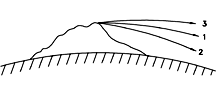# (21) Kepler's Third LawIndex18c. Work18d. Work against
Electric Forces19.Motion in a Circle20. Newton's Gravity21. Kepler's 3rd Law21a.Applying 3rd Law21b. Fly to Mars! (1)21c. Fly to Mars! (2)21d. Fly to Mars! (3)22.Reference Frames22a.Starlight Aberration22b. Relativity22c. Flight (1)22d. Flight (2)

Besides the Moon, Earth now has many artificial satellites, put up by us earthlings for a variety of purposes. The calculation applied by Newton to the Moon can also be used for them.

## Orbital Velocity

Suppose the Earth were a perfect sphere of radius 1 RE = 6 317 000 meters and had no atmosphere. In principle, a satellite could then orbit just above its surface. The Earth would pull it downwards with a force F = mg, and because of the direction of this force, any accelerations would be in the up-down direction, too.

If the satellite is in a stable circular orbit and its velocity is V, then F supplies just the right amount of pull to keep the motion going. Which means

mg = F = mV2/RE

Dividing both sides by m shows that the mass of the satellite does not matter, and leaves

V2/RE = g

Multiplication of both sides by RE: gives

V2 = (g) (RE) = (9.81) (6 371 000) = 62 499 510 (m2/sec2)

A square root is traditionally denoted by the symbol √ . The square root of 2, for instance, can be written √ 2 = 1.41412… and so

V = √(g RE) = 7905 m/sec = 7.905 km/s = VoThis is the velocity required by the satellite to stay in its orbit ("1" in the drawing). Any slower and it loses altitude and hits the Earth ("2"), any faster and it rises to greater distance ("3"). For comparison, a jetliner flies at about 250 m/sec, a rifle bullet at about 600 m/sec.

If the speed V of our satellite is only moderately greater than Vo curve "3" will be part of a Keplerian ellipse and will ultimately turn back towards Earth. If however V is greater than 1. 4142. . .times Vo the satellite has attained escape velocity and will never come back: this comes to about 11.2 km/sec.

The value of 11.2 km/s was already derived in the section on Kepler's 2nd law, where the expression for the energy of Keplerian motion was given (without proof) as

E = 1/2 mv2 – k m/r

where for a satellite orbiting Earth at distance of one Earth radius RE, the constant k equals k=gRE2. The energy is negative for any spacecraft captured by Earth's gravity, positive for any not held captive, and zero for one just escaping. Let V1 be the velocity of such a spacecraft, located at distance RE but with zero energy, i.e. E=0. Then

(1/2) m V12 = mgRE
from which
V12 = 2gRE
which gives, as claimed,
V1= 1.414..V0

## Kepler's Third Law for Earth Satellites

The velocity for a circular Earth orbit at any other distance r is similarly calculated, but one must take into account that the force of gravity is weaker at greater distances, by a factor (RE/r)2. We then get

V2/r = g (RE r)2 = g RE2/r2

Let T be the orbital period, in seconds. Then (as noted earlier), the distance 2 πr covered in one orbit equals VT.

>
 VT = 2 π r V = 2 π r/T V2 = 4 π2r2/T2 V2/r = 4 π2 r/T2

and by the earlier equality

4 π2r/T2 = g RE2/r2

Get rid of fractions by multiplying both sides by r2T2

4 π2r3 = g RE2 T2

To better see what we have, divide both sides by g RE2, isolating T2:

T2 = (4 π2/g RE2) r3

What's inside the brackets is just a number. The rest tells a simple message--T2 is proportional to r3, the orbital period squared is proportional to the distance cubes. This is Kepler's 3rd law, for the special case of circular orbits around Earth.

If you are not yet tired of the calculation, you may click here for turning the above into a practical formula.

### Tidbit

The first claim of escape from Earth' gravity goes back to 1634. Marin Mersenne, a friend of Descartes, got involved in an arcane argument: if a cannon barrel were set into the ground precisely vertically and fired, would the cannonball fall back into the barrel or not? Mersenne and a friend of his named Petit, with ties to the military, obtained a cannon and actually tried it out. (My source did not mention how close to the cannon they stood at that time.)

The result was somewhat unexpected: the cannon ball was never found again! Frustrated, they consulted Descartes, whose opinion was that the projectile went so far from Earth that it escaped its pull and never came back.
(""Retombera-t-il?", Amer. J. of Physics 22, p.76, 1954)
Questions from Users:   Spaceflight without escape velocity?
***     Does the solar wind have escape velocity?
***     Is orbital motion same as free fall?

Deriving a practical formula from Kepler's 3rd law.

Next Regular Stop: Frames of Reference: The Basics

Author and Curator:   Dr. David P. Stern
Mail to Dr.Stern:   stargaze("at" symbol)phy6.org .

Last updated: 5-20-08